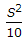# Civil Engineering - Highway Engineering

### Exercise :: Highway Engineering - Section 2

26.

Road width 8.8 m of two lane National highways or State highways in mountainous terrain

 A. excludes the width of parapet (0.6 m) B. excludes the width of side drain (0.6 m) C. excludes the width of parapet and side drain D. includes the width of parapet and side drain

Explanation:

No answer description available for this question. Let us discuss.

27.

Pick up the correct statement from the following:

 A. Long tangent sections exceeding 3 km in length should be avoided B. Curve length should be at least 150 metres for a deflection angle of 5 degress C. For every degree decrease in the deflection angle, 30 metre length of curve to be increased D. If the deflection angle is less than 1°, no curve is designed E. All the above.

Explanation:

No answer description available for this question. Let us discuss.

28.

Transverse joints are provided at distances varying from

 A. 10 m to 15 m B. 12 m to 18 m C. 16 m to 24 m D. 17 m to 27 m E. 25 m to 35 m

Explanation:

No answer description available for this question. Let us discuss.

29.

If N is the algebraic difference of grades, S is the minimum sight distance in metres, the length (L) of a summit curve is

 A.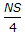B.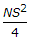C.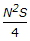D.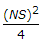E. none of these.

Explanation:

No answer description available for this question. Let us discuss.

30.

If N is the net difference of grades, S is the minimum overtaken sight distance in metres, the length (L) of a summit curve, is

 A.B.C.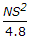D.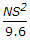E.